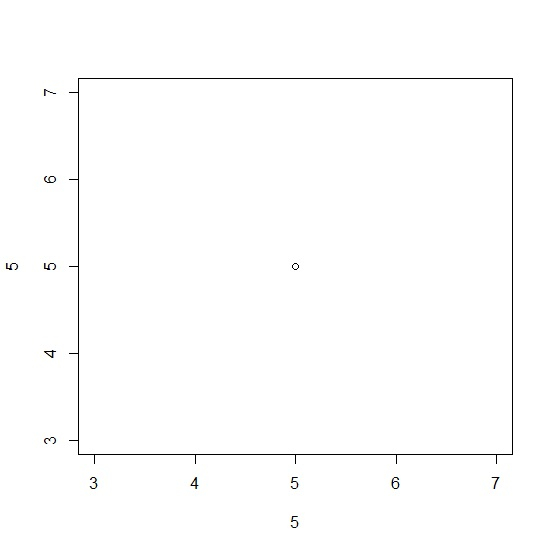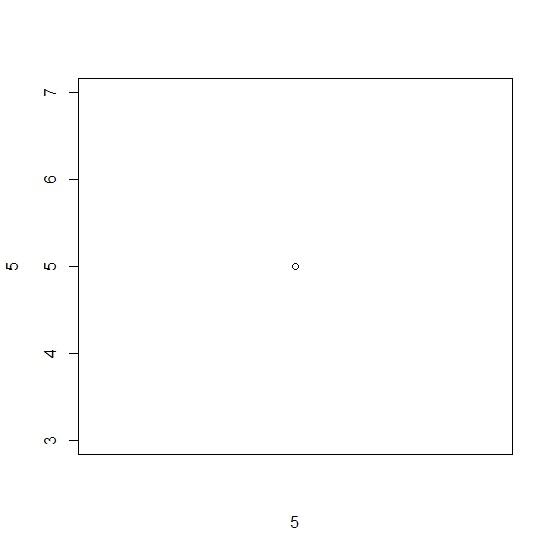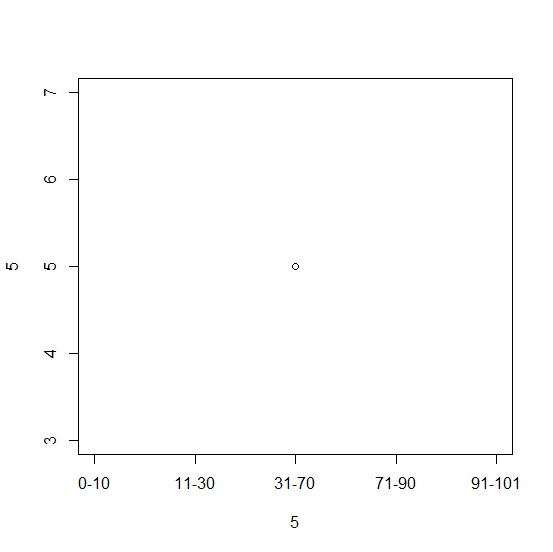# How to display X-axis labels with dash in base R plot?

To display X-axis labels with dash in base R plot, we can use axis function and define the labels manually. For this purpose, we can first create the plot without X-axis labels by using the argument xaxt="n" and then use the axis function.

Check out the below example to understand how it can be done.

## Example

In order to display X-axis labels with dash in base R plot, use the following code −

plot(5,5)

## Output

If you execute all the above given codes as a single program, it generates the following output −In order to display X-axis labels with dash in base R plot, add the following code to the above code −

plot(5,5,xaxt="n")


## Output

If you execute all the above given codes as a single program, it generates the following output −In order to display X-axis labels with dash in base R plot, add the following code to the above code −

plot(5,5,xaxt="n")
axis(1,at=c(3:7),labels=c("0-10","11-30","31-70","71-90","91-101"))

## Output

If you execute all the above given codes as a single program, it generates the following output −